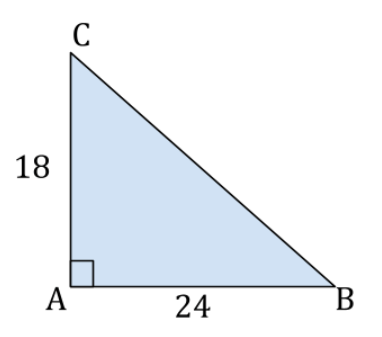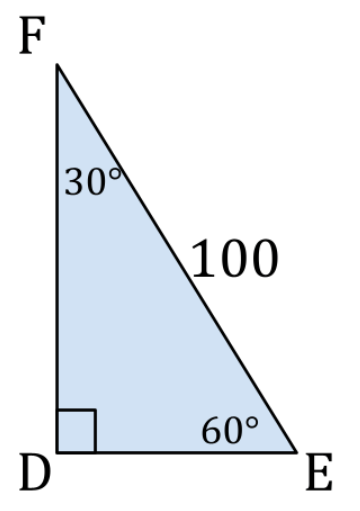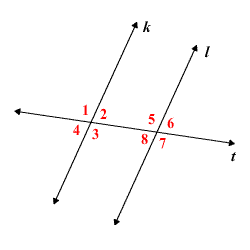Strategy
Circles
Triangles
Potpourri
100

This is the first action you should take if you see a geometry problem without any kind of picture or figure.

What is DRAW?

100

This is the total distance around the figure, found by adding all of the side lengths.

What is perimeter?

100

This is the measurement of the total distance around the edge of a circle.

What is circumference?

100

This is the term for a triangle in which all three sides are equal.

What is an equilateral triangle?

100

This is the number of degrees that supplementary angles add up to.

What is 180?

200

Writing down these with your pencil will prevent silly mistakes and help you to see how to approach harder problems.

What are formulas?

200

This is the formula used to calculate area.

What is  A=lw or  A=bh ?

200

This is the word used for ANY line segment from the center of a circle to a point on the circle.

200

This is the formula used to calculate the area of a triangle.

What is  A=1/2bh ?

200

This is the word used when one figure is placed inside another.

What is inscribed?

300

This strategy is used when there are variables or letters in the answer choices, or if you have an "always," "sometimes," or "never" question.

What is Plugging In?

300

This is a quadrilateral with four equal sides AND four right angles.

What is a square?

300

This is the formula for calculating circumference.

What is  C=2pir or  C=pid ?

300

This is the Pythagorean theorem, which shows the relationship between the lengths of the sides of a right triangle.

What is  a^2+b^2=c^2 ?

300

Opposite angles formed at the intersection of two lines, called vertical angles, are related in this way.

What is equal?

400

This is the strategy that can be used on ANY question, but is best for those asking "how much" or "how many" with answer choices around in order from smallest to biggest (or vice versa).

What is PITA (Plugging In The Answers)?

400

This is a quadrilateral with only two sides that are parallel.

What is a trapezoid?

400

This is the formula for calculating the area of a circle.

What is  A=pir^2 ?

400

The length of the hypotenuse of triangle ABC:What is 30?

400

This word means to divide any angle, line, or segment into two identical pieces.

What is bisect?

500

These can be used for any problem with ratios, percents, rates, unit conversions, or other "relationship numbers."

What are proportions or (ratio) charts?

500

This is the sum the four interior angles of a quadrilateral.

What is a 360 degrees?

500

This is the radius of a circle that has an area of 8𝜋 (SIMPLIFIED).

What is  2sqrt(2) ?

500

The length of side DF in the special right triangle DEF:What is  50sqrt(3) ?

500

The measurement, in degrees, of angle 7 below if angle 1 is 105 degrees and lines k and l are parallel.What is 105 degrees?

Click to zoom